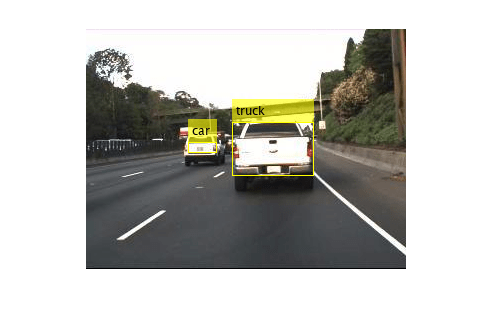# predict

Compute YOLO v3 deep learning network outputs for inference

## Syntax

``output = predict(detector,dlX)``

## Description

example

````output = predict(detector,dlX)` computes the YOLO v3 deep learning network outputs during inference, given the detector and the test data. Use this function to get predictions from the output layers of the YOLO v3 deep learning network during inference. NoteTo run this function, you will require the Deep Learning Toolbox™. ```

## Examples

collapse all

Load a pretrained YOLO v3 object detector.

`detector = yolov3ObjectDetector('tiny-yolov3-coco');`

Load the test image for prediction.

`I = imread('highway.png');`

Preprocess the test image and convert the preprocessed image to a formatted dlarray object.

```[Ip,info] = preprocess(detector,I); Ip = im2single(Ip); dlX = dlarray(Ip,'SSCB');```

Compute predictions for the test image. The `predict` function returns the predictions for the feature maps from the output layers of the YOLO v3 deep learning network. The first column contains the confidence scores. Columns 2 to 5 contain the bounding box locations computed relative to the grid cell coordinates. The sixth column contains the class probabilities for each class used during training. The seventh and the eighth column contains the prior width and prior height of bounding boxes as computed by the network, respectively.

`output = predict(detector,dlX)`
```output=2×8 cell array Columns 1 through 3 {13x13x3x1 dlarray} {13x13x3x1 dlarray} {13x13x3x1 dlarray} {26x26x3x1 dlarray} {26x26x3x1 dlarray} {26x26x3x1 dlarray} Columns 4 through 6 {13x13x3x1 dlarray} {13x13x3x1 dlarray} {13x13x240x1 dlarray} {26x26x3x1 dlarray} {26x26x3x1 dlarray} {26x26x240x1 dlarray} Columns 7 through 8 {13x13x3x1 dlarray} {13x13x3x1 dlarray} {26x26x3x1 dlarray} {26x26x3x1 dlarray} ```

You can then get the final detections by using the predictions for features with maximum objectness scores. The objectness score is the product of confidence score and class probability. To compute the exact bounding box location, you must map the predicted bounding box values to box coordinates. Alternatively, you can use the `detect` function to directly get the detection results. The `detect` function internally calls the `predict` function to compute the feature maps.## Input Arguments

collapse all

YOLO v3 object detector, specified as a `yolov3ObjectDetector` object.

Test data, specified as a formatted `dlarray` (Deep Learning Toolbox) object. The test data can contain one or more test images.

## Output Arguments

collapse all

Output predictions, returned as an N-by-8 cell array of formatted `dlarray` (Deep Learning Toolbox) objects. N is the number of output layers in the YOLO v3 deep learning network. Each row in the cell array is of form [conf bx by bw bh prob tw th]. The function returns predictions as a formatted `dlarray` (Deep Learning Toolbox) value..

 Predictions Description conf Confidence scores for each bounding box. bx X-coordinate of the center of the predicted bounding box relative to the location of the grid cell. by Y-coordinate of the center of the predicted bounding box relative to the location of the grid cell. bw Width of the predicted bounding box relative to the location of the grid cell. bh Height of the predicted bounding boxes relative to the location of the grid cell. prob Class probabilities predicted for each feature in the output feature map. tw Prior width of the bounding boxes as computed by the network. th Prior height of the bounding boxes as computed by the network.

## Version History

Introduced in R2021a